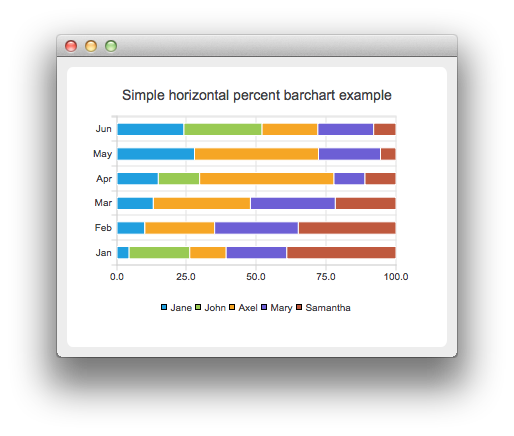# Creating Horizontal Percent Bar Charts

Note: This is part of the Charts with Widgets Gallery example.

The horizontal percent bar chart shows the data in a set as a percentage of all sets per category.

Creating a horizontal percent bar chart is just like creating a regular bar chart, except that for a horizontal percent bar chart, we use the QHorizontalPercentBarSeries api instead of QBarSeries. Also, in the bar chart, we used a nice numbers algorithm to make the y-axis numbering look better. With the percent bar chart there is no need for that, because the maximum y-axis value is always 100.The barsets are used in same way in all barcharts. To illustrate the difference between various barcharts, we use same data in examples. The data which the bar chart visualizes is defined by QBarSet instances. Here we create the sets and append data to them. The data is appended here with << operator. Alternatively the append method could be used.

```auto set0 = new QBarSet("Jane");
auto set1 = new QBarSet("John");
auto set2 = new QBarSet("Axel");
auto set3 = new QBarSet("Mary");
auto set4 = new QBarSet("Samantha");

*set0 << 1 << 2 << 3 << 4 << 5 << 6;
*set1 << 5 << 0 << 0 << 4 << 0 << 7;
*set2 << 3 << 5 << 8 << 13 << 8 << 5;
*set3 << 5 << 6 << 7 << 3 << 4 << 5;
*set4 << 9 << 7 << 5 << 3 << 1 << 2;```

We create the series and append the bar sets to it. The series takes ownership of the barsets. The series groups the data from sets to categories. The first values of each set are grouped together in the first category, the second value in the second category etc.

```auto series = new QHorizontalPercentBarSeries;
series->append(set0);
series->append(set1);
series->append(set2);
series->append(set3);
series->append(set4);```

Here we create the chart object and add the series to it. We set the title for chart with setTitle and then turn on animations of the series by calling setAnimationOptions(QChart::SeriesAnimations)

```auto chart = new QChart;
chart->setTitle("Simple Horizontal Percent Bar Chart");
chart->setAnimationOptions(QChart::SeriesAnimations);```

To have the categories displayed on an axis, we need to create a QBarCategoryAxis first. Here we create a category axis with a list of categories and add it to the chart on the left-hand side, acting as the y-axis. The chart takes ownership of the axis. For x-axis we use a value axis, aligned to the bottom.

```QStringList categories {"Jan", "Feb", "Mar", "Apr", "May", "Jun"};
auto axisY = new QBarCategoryAxis;
axisY->append(categories);
series->attachAxis(axisY);
auto axisX = new QValueAxis;
```chart->legend()->setVisible(true);
`createDefaultChartView(chart);`